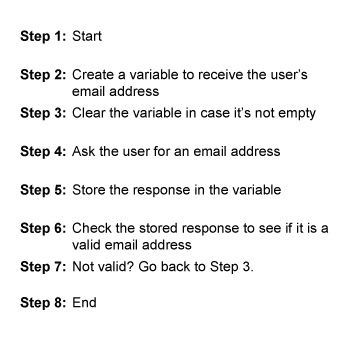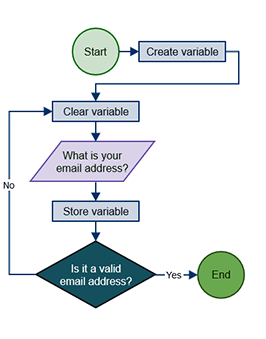## Saturday, June 1, 2019

### Algorithms for Every One

What is "Algorithms"?

A process or set of rules to be followed in calculations or other problem-solving operations, especially by a computer.

## What Is a Programming Algorithm?

So, what is a programming algorithm? You can think of a programming algorithm as a recipe that describes the exact steps needed for the computer to solve a problem or reach a goal. We've all seen food recipes - they list the ingredients needed and a set of steps for how to make the described meal. Well, an algorithm is just like that. In computer lingo, the word for a recipe is a procedure, and the ingredients are called inputs. Your computer looks at your procedure, follows it to the letter, and you get to see the results, which are called outputs. A programming algorithm describes how to do something, and your computer will do it exactly that way every time. Well, it will once you convert your algorithm into a language it understands!
However, it's important to note that a programming algorithm is not computer code. It's written in simple English (or whatever the programmer speaks). It doesn't beat around the bush--it has a start, a middle, and an end. In fact, you will probably label the first step 'start' and the last step 'end.' It includes only what you need to carry out the task. It does not include anything unclear, often called ambiguous in computer lingo, that someone reading it might wonder about.
It always leads to a solution and tries to be the most efficient solution we can think up. It's often a good idea to number the steps, but you don't have to. Instead of numbered steps, some folks use indentation and write in pseudocode, which is a semi-programming language used to describe the steps in an algorithm. But, we won't use that here since simplicity is the main thing. Other folks just use a diagram called a flowchart, which we will discuss soon.

## Programming Algorithm Example

Okay, you probably wish you could see an example, right? So, what exactly does an algorithm in programming look like? Well, asking a user for an email address is probably one of the most common tasks a web-based program might need to do, so that is what we will use here for an example. An algorithm can be written as a list of steps using text or as a picture with shapes and arrows called a flowchart. We will make one of each which you will see here:Wasn't that easy? Notice how the top of our example is just a numbered list of steps using plain English, stating exactly what we want the procedure to do (no more, no less). The bottom is the very same algorithm, but this time, we used shapes and arrows in a flowchart (like a map of the route), so that a reader can visualize the journey. That's a nice thing here, because in one of our steps (step 7) a decision must be made and, depending on the result of that decision, our steps may not go in order from start to end.
Okay! Let's take a quick run through our little recipe:
1. Step 1 is really just a reminder that this is a procedure with a beginning and an end.
2. In step 2, we make a place in the computer to store what the user types in, also called a variable
3. In step 3, we clear this variable because we might need to use it again and don't want the old contents mixed in with the new.
4. In step 4, we prompt the user for an email address
5. In step 5, we stick it in our nifty variable.
6. In step 6, we tell our computer to take a close look at this email address-- is it really an email address?

## Qualities of a good algorithm

1. Input and output should be defined precisely.
2. Each steps in algorithm should be clear and unambiguous.
3. Algorithm should be most effective among many different ways to solve a problem.
4. An algorithm shouldn't have computer code. Instead, the algorithm should be written in such a way that, it can be used in similar programming languages.

## Examples Of Algorithms In Programming

```Step 1: Start
Step 2: Declare variables num1, num2 and sum.
Step 3: Read values num1 and num2.
Step 4: Add num1 and num2 and assign the result to sum.
sum←num1+num2
Step 5: Display sum
Step 6: Stop
```

```Step 1: Start
Step 2: Declare variables a,b and c.
Step 3: Read variables a,b and c.
Step 4: If a>b
If a>c
Display a is the largest number.
Else
Display c is the largest number.
Else
If b>c
Display b is the largest number.
Else
Display c is the greatest number.
Step 5: Stop
```

Write an algorithm to find all roots of a quadratic equation ax2+bx+c=0.

```Step 1: Start
Step 2: Declare variables a, b, c, D, x1, x2, rp and ip;
Step 3: Calculate discriminant
D←b2-4ac
Step 4: If D≥0
r1←(-b+√D)/2a
r2←(-b-√D)/2a
Display r1 and r2 as roots.
Else
Calculate real part and imaginary part
rp←b/2a
ip←√(-D)/2a
Display rp+j(ip) and rp-j(ip) as roots
Step 5: Stop
```